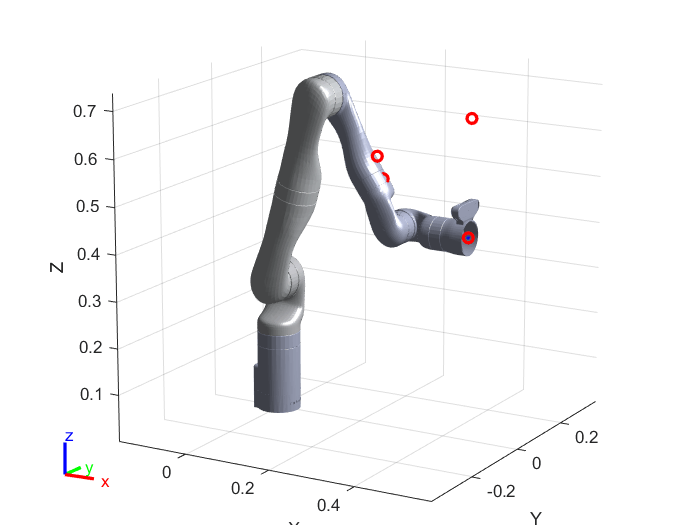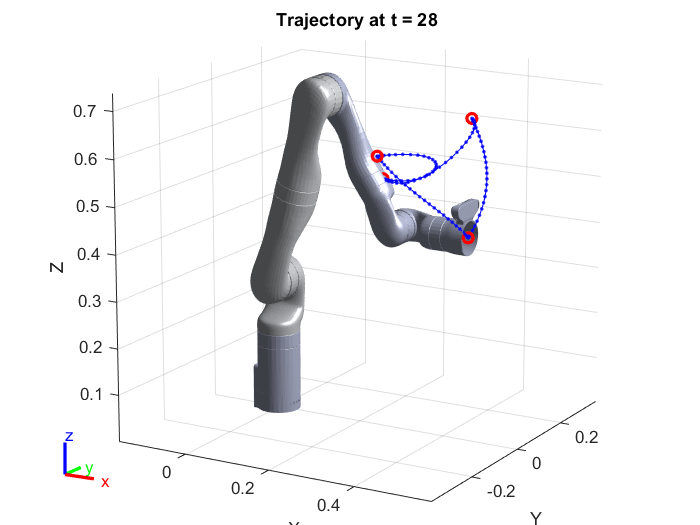# Generate a Trajectory Using a Set of Waypoints for KINOVA Gen3 Robot End-Effector

This example shows how to generate and interpolate trajectories from a set of waypoints. This example covers common ways of generating trajectories for robot arms such as cubic polynomials, quintic polynomials, and trapezoidal trajectories.

Before proceeding further, see the example Track Pre-Computed Trajectory of KINOVA Gen3 Robot End-Effector Using Inverse Kinematics and KINOVA KORTEX MATLAB API. This provides you information on inverse kinematic for robotic manipulators and sending commands to Kinova Gen3 robot to track pre-computed trajectories.

Clear all output in the live script by right clicking anywhere in the live script and then clicking ‘Clear All Output’ or navigate to VIEW > Clear all Output.

### Create the Robot Model

Create a Rigid Body Tree for Gen3 robot, set the home configuration and calculate the transformation at the home configuration:

```gen3 = loadrobot("kinovaGen3"); gen3.DataFormat = 'column'; q_home = [0 15 180 -130 0 55 90]'*pi/180; eeName = 'EndEffector_Link'; T_home = getTransform(gen3, q_home, eeName); T_home(1:4,4) = [0;0;0;1];```

### Visualize the robot at home configuration

```show(gen3,q_home); axis auto; view([60,10]); ```### Define a Set of Waypoints Based on the Tool Position

```toolPositionHome = [0.455 0.001 0.434]; waypoints = toolPositionHome' + ... [0 0 0 ; -0.1 0.2 0.2 ; -0.2 0 0.1 ; -0.1 -0.2 0.2 ; 0 0 0]';```

Define orientation

The orientation is represented in Euler angles and the convention used is the Tait-Bryan, extrinsic ZYX.

```orientations = [pi/2 0 pi/2; (pi/2)+(pi/4) 0 pi/2; pi/2 0 pi; (pi/2)-(pi/4) 0 pi/2; pi/2 0 pi/2]';```

Define array of waypoint times

```waypointTimes = 0:7:28; ts = 0.25; trajTimes = 0:ts:waypointTimes(end);```

Define boundary conditions for velocity and acceleration

```waypointVels = 0.1 *[ 0 1 0; -1 0 0; 0 -1 0; 1 0 0; 0 1 0]'; waypointAccels = zeros(size(waypointVels)); waypointAccelTimes = diff(waypointTimes)/4;```

### Create Inverse Kinematics Solver and Set Parameters

```ik = inverseKinematics('RigidBodyTree',gen3); ikWeights = [1 1 1 1 1 1]; ikInitGuess = q_home'; ikInitGuess(ikInitGuess > pi) = ikInitGuess(ikInitGuess > pi) - 2*pi; ikInitGuess(ikInitGuess < -pi) = ikInitGuess(ikInitGuess < -pi) + 2*pi;```

### Set Plot and Display Waypoints

```plotMode = 1; % 0 = None, 1 = Trajectory, 2 = Coordinate Frames show(gen3,q_home,'Frames','off','PreservePlot',false); hold on if plotMode == 1 hTraj = plot3(waypoints(1,1),waypoints(2,1),waypoints(3,1),'b.-'); end plot3(waypoints(1,:),waypoints(2,:),waypoints(3,:),'ro','LineWidth',2); axis auto; view([30 15]);```### Solve the Inverse Kinematics for Each Waypoint

Set includeOrientation to zero if you do not want to define different orientation at different waypoints. When includeOrientation is set to false, a default orientation is used to compute the trajectory.

```includeOrientation = true; numWaypoints = size(waypoints,2); numJoints = numel(gen3.homeConfiguration); jointWaypoints = zeros(numJoints,numWaypoints); for idx = 1:numWaypoints if includeOrientation tgtPose = trvec2tform(waypoints(:,idx)') * eul2tform(orientations(:,idx)'); else tgtPose = trvec2tform(waypoints(:,idx)') * eul2tform([pi/2 0 pi/2]); %#ok<UNRCH> end [config,info] = ik(eeName,tgtPose,ikWeights',ikInitGuess'); jointWaypoints(:,idx) = config'; end```

### Generate a Trajectory in Joint Space using Interpolation

Select method of interpolation from trapezoidal velocity profiles, third-order polynomial, fifth-order polynomial or B-spline polynomial by modifying the variable trajType.

```trajType = 'trap'; switch trajType case 'trap' [q,qd,qdd] = trapveltraj(jointWaypoints,numel(trajTimes), ... 'AccelTime',repmat(waypointAccelTimes,[numJoints 1]), ... 'EndTime',repmat(diff(waypointTimes),[numJoints 1])); case 'cubic' [q,qd,qdd] = cubicpolytraj(jointWaypoints,waypointTimes,trajTimes, ... 'VelocityBoundaryCondition',zeros(numJoints,numWaypoints)); case 'quintic' [q,qd,qdd] = quinticpolytraj(jointWaypoints,waypointTimes,trajTimes, ... 'VelocityBoundaryCondition',zeros(numJoints,numWaypoints), ... 'AccelerationBoundaryCondition',zeros(numJoints,numWaypoints)); case 'bspline' ctrlpoints = jointWaypoints; % Can adapt this as needed [q,qd,qdd] = bsplinepolytraj(ctrlpoints,waypointTimes([1 end]),trajTimes); otherwise error('Invalid trajectory type! Use ''trap'', ''cubic'', ''quintic'', or ''bspline'''); end```

### Visualize the Solution

```for idx = 1:numel(trajTimes) config = q(:,idx)'; % Find Cartesian points for visualization eeTform = getTransform(gen3,config',eeName); if plotMode == 1 eePos = tform2trvec(eeTform); set(hTraj,'xdata',[hTraj.XData eePos(1)], ... 'ydata',[hTraj.YData eePos(2)], ... 'zdata',[hTraj.ZData eePos(3)]); elseif plotMode == 2 plotTransforms(tform2trvec(eeTform),tform2quat(eeTform),'FrameSize',0.05); end % Show the robot show(gen3,config','Frames','off','PreservePlot',false); title(['Trajectory at t = ' num2str(trajTimes(idx))]) drawnow end```### Send the Trajectory to the Hardware

Expected Motion of the robot (Assuming you are starting from the retract position)Press ‘y’ and hit enter if you want to command the Kinova Gen3 robot to track the calculated trajectory or hit enter to finish the example

```prompt = 'Do you want to send same trajectory to the hardware? y/n [n]: '; str = input(prompt,'s'); if isempty(str) str = 'n'; end if str == 'n' clear; return; end```

Command Kinova Gen3 robot to track the pre-computed trajectory

```q = q*180/pi; qd = qd*180/pi; qdd = qdd*180/pi; timestamp = (0:0.001:waypointTimes(end))'; qs_deg = interp1(trajTimes',q',timestamp); vel = interp1(trajTimes',qd',timestamp); acc = interp1(trajTimes',qdd',timestamp);```

Connect to the Robot

```Simulink.importExternalCTypes(which('kortex_wrapper_data.h')); gen3Kinova = kortex(); gen3Kinova.ip_address = '192.168.1.10'; gen3API.user = 'admin'; gen3API.password = 'admin'; isOk = gen3Kinova.CreateRobotApisWrapper(); if isOk disp('You are connected to the robot!'); else error('Failed to establish a valid connection!'); end```

Visualize the actual movement of the robot

```title('Actual Movement of the Robot'); [~,~, actuatorFb, ~] = gen3Kinova.SendRefreshFeedback(); show(gen3, ((actuatorFb.position)*pi/180)','Frames','off','PreservePlot',false); drawnow;```

### Send Robot to Starting Point of the Trajectory

```jointCmd = wrapTo360(qs_deg(1,:)); constraintType = int32(0); speed = 0; duration = 0; isOk = gen3Kinova.SendJointAngles(jointCmd, constraintType, speed, duration); if isOk disp('success'); else disp('SendJointAngles cmd error'); return; end```

Check if the robot has reached the starting position

```while 1 [isOk,~, actuatorFb, ~] = gen3Kinova.SendRefreshFeedback(); show(gen3, ((actuatorFb.position)*pi/180)','Frames','off','PreservePlot',false); drawnow; if isOk if max(abs(wrapTo360(qs_deg(1,:))-actuatorFb.position)) < 0.1 disp('Starting point reached.') break; end else error('SendRefreshFeedback error') end end```

### Send Pre-Computed Trajectory

```isOk = gen3Kinova.SendPreComputedTrajectory(qs_deg.', vel.', acc.', timestamp.', size(timestamp,1)); if isOk disp('SendPreComputedTrajectory success'); else disp('SendPreComputedTrajectory cmd error'); end```

Check if the robot has reached the end position

```status = 1; %% Check if the robot has reached the end postion while status [isOk,~, actuatorFb, ~] = gen3Kinova.SendRefreshFeedback(); show(gen3, ((actuatorFb.position)*pi/180)','Frames','off','PreservePlot',false); drawnow; if isOk [~,status] = gen3Kinova.GetMovementStatus(); else error('SendRefreshFeedback error'); end end disp('Reached to Final waypoint.');```

### Disconnect from the Robot

Use this command to disconnect from Kinova Gen3 robot Robot.

`isOk = gen3Kinova.DestroyRobotApisWrapper();`

Clear Workspace

`clear;`